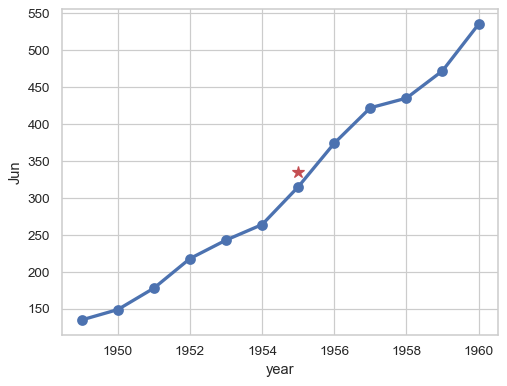# seaborn.pointplot#

seaborn.pointplot(data=None, *, x=None, y=None, hue=None, order=None, hue_order=None, estimator='mean', errorbar=('ci', 95), n_boot=1000, units=None, seed=None, color=None, palette=None, hue_norm=None, markers=<default>, linestyles=<default>, dodge=False, log_scale=None, native_scale=False, orient=None, capsize=0, formatter=None, legend='auto', err_kws=None, ci=<deprecated>, errwidth=<deprecated>, join=<deprecated>, scale=<deprecated>, ax=None, **kwargs)#

Show point estimates and errors using lines with markers.

A point plot represents an estimate of central tendency for a numeric variable by the position of the dot and provides some indication of the uncertainty around that estimate using error bars.

Point plots can be more useful than bar plots for focusing comparisons between different levels of one or more categorical variables. They are particularly adept at showing interactions: how the relationship between levels of one categorical variable changes across levels of a second categorical variable. The lines that join each point from the same `hue` level allow interactions to be judged by differences in slope, which is easier for the eyes than comparing the heights of several groups of points or bars.

Note

By default, this function treats one of the variables as categorical and draws data at ordinal positions (0, 1, … n) on the relevant axis. As of version 0.13.0, this can be disabled by setting `native_scale=True`.

Parameters:
dataDataFrame, Series, dict, array, or list of arrays

Dataset for plotting. If `x` and `y` are absent, this is interpreted as wide-form. Otherwise it is expected to be long-form.

x, y, huenames of variables in `data` or vector data

Inputs for plotting long-form data. See examples for interpretation.

order, hue_orderlists of strings

Order to plot the categorical levels in; otherwise the levels are inferred from the data objects.

estimatorstring or callable that maps vector -> scalar

Statistical function to estimate within each categorical bin.

errorbarstring, (string, number) tuple, callable or None

Name of errorbar method (either “ci”, “pi”, “se”, or “sd”), or a tuple with a method name and a level parameter, or a function that maps from a vector to a (min, max) interval, or None to hide errorbar.

New in version v0.12.0.

n_bootint

Number of bootstrap samples used to compute confidence intervals.

unitsname of variable in `data` or vector data

Identifier of sampling units; used by the errorbar function to perform a multilevel bootstrap and account for repeated measures

seedint, `numpy.random.Generator`, or `numpy.random.RandomState`

Seed or random number generator for reproducible bootstrapping.

colormatplotlib color

Single color for the elements in the plot.

palettepalette name, list, or dict

Colors to use for the different levels of the `hue` variable. Should be something that can be interpreted by `color_palette()`, or a dictionary mapping hue levels to matplotlib colors.

Markers to use for each of the `hue` levels.

linestylesstring or list of strings

Line styles to use for each of the `hue` levels.

dodgebool or float

Amount to separate the points for each level of the `hue` variable along the categorical axis. Setting to `True` will apply a small default.

log_scalebool or number, or pair of bools or numbers

Set axis scale(s) to log. A single value sets the data axis for any numeric axes in the plot. A pair of values sets each axis independently. Numeric values are interpreted as the desired base (default 10). When `None` or `False`, seaborn defers to the existing Axes scale.

New in version v0.13.0.

native_scalebool

When True, numeric or datetime values on the categorical axis will maintain their original scaling rather than being converted to fixed indices.

New in version v0.13.0.

orient“v” | “h” | “x” | “y”

Orientation of the plot (vertical or horizontal). This is usually inferred based on the type of the input variables, but it can be used to resolve ambiguity when both `x` and `y` are numeric or when plotting wide-form data.

Changed in version v0.13.0: Added ‘x’/’y’ as options, equivalent to ‘v’/’h’.

capsizefloat

Width of the “caps” on error bars, relative to bar spacing.

formattercallable

Function for converting categorical data into strings. Affects both grouping and tick labels.

New in version v0.13.0.

legend“auto”, “brief”, “full”, or False

How to draw the legend. If “brief”, numeric `hue` and `size` variables will be represented with a sample of evenly spaced values. If “full”, every group will get an entry in the legend. If “auto”, choose between brief or full representation based on number of levels. If `False`, no legend data is added and no legend is drawn.

New in version v0.13.0.

err_kwsdict

Parameters of `matplotlib.lines.Line2D`, for the error bar artists.

New in version v0.13.0.

cifloat

Level of the confidence interval to show, in [0, 100].

Deprecated since version v0.12.0: Use `errorbar=("ci", ...)`.

errwidthfloat

Thickness of error bar lines (and caps), in points.

Deprecated since version 0.13.0: Use `err_kws={'linewidth': ...}`.

joinbool

If `True`, connect point estimates with a line.

Deprecated since version v0.13.0: Set `linestyle="none"` to remove the lines between the points.

scalefloat

Scale factor for the plot elements.

Deprecated since version v0.13.0: Control element sizes with `matplotlib.lines.Line2D` parameters.

axmatplotlib Axes

Axes object to draw the plot onto, otherwise uses the current Axes.

kwargskey, value mappings

Other parameters are passed through to `matplotlib.lines.Line2D`.

New in version v0.13.0.

Returns:
axmatplotlib Axes

Returns the Axes object with the plot drawn onto it.

`barplot`

Show point estimates and confidence intervals using bars.

`catplot`

Combine a categorical plot with a `FacetGrid`.

Notes

It is important to keep in mind that a point plot shows only the mean (or other estimator) value, but in many cases it may be more informative to show the distribution of values at each level of the categorical variables. In that case, other approaches such as a box or violin plot may be more appropriate.

Examples

Group by a categorical varaible and plot aggregated values, with confidence intervals:

```sns.pointplot(data=penguins, x="island", y="body_mass_g")
```Add a second layer of grouping and differentiate with color:

```sns.pointplot(data=penguins, x="island", y="body_mass_g", hue="sex")
```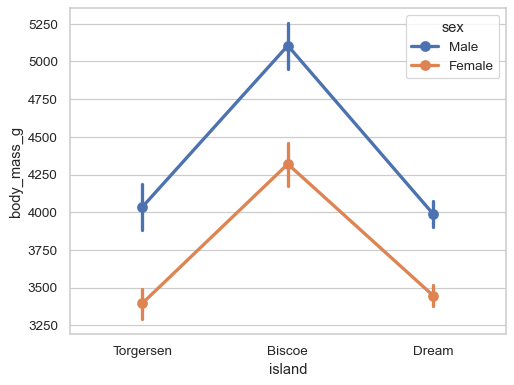Redundantly code the `hue` variable using the markers and linestyles for better accessibility:

```sns.pointplot(
data=penguins,
x="island", y="body_mass_g", hue="sex",
markers=["o", "s"], linestyles=["-", "--"],
)
```Use the error bars to represent the standard deviation of each distribution:

```sns.pointplot(data=penguins, x="island", y="body_mass_g", errorbar="sd")
```Customize the appearance of the plot:

```sns.pointplot(
data=penguins, x="body_mass_g", y="island",
errorbar=("pi", 100), capsize=.4,
color=".5", linestyle="none", marker="D",
)
```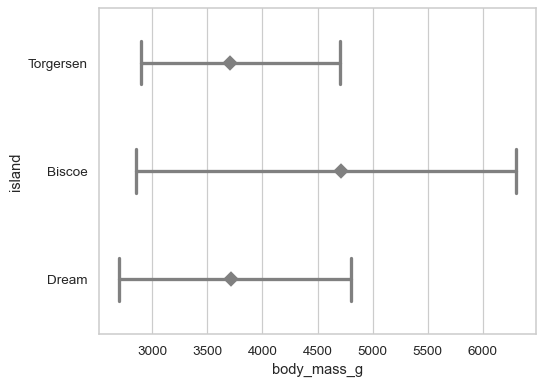“Dodge” the artists along the categorical axis to reduce overplotting:

```sns.pointplot(data=penguins, x="sex", y="bill_depth_mm", hue="species", dodge=True)
```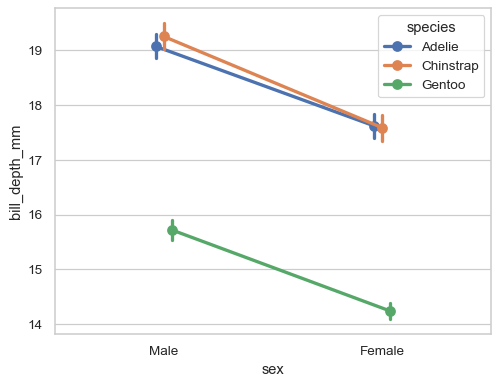Dodge by a specific amount, relative to the width alloted for each level:

```sns.stripplot(
data=penguins, x="species", y="bill_depth_mm", hue="sex",
dodge=True, alpha=.2, legend=False,
)
sns.pointplot(
data=penguins, x="species", y="bill_depth_mm", hue="sex",
dodge=.4, linestyle="none", errorbar=None,
marker="_", markersize=20, markeredgewidth=3,
)
```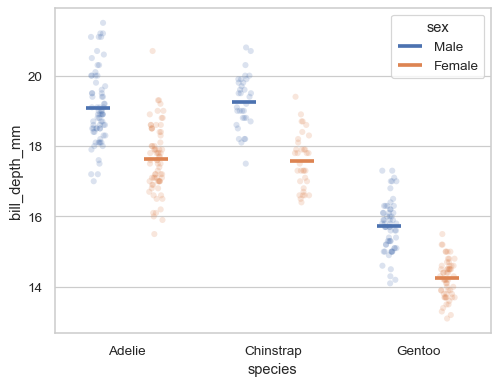When variables are not explicity assigned and the dataset is two-dimensional, the plot will aggregate over each column:

```flights_wide = flights.pivot(index="year", columns="month", values="passengers")
sns.pointplot(flights_wide)
```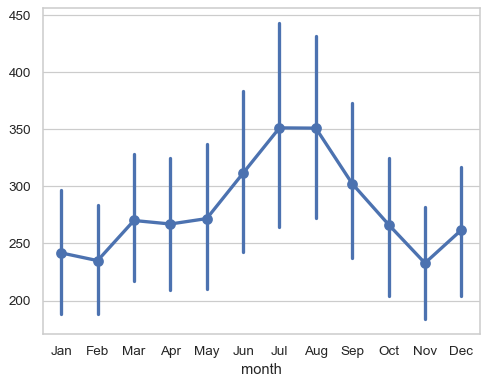With one-dimensional data, each value is plotted (relative to its key or index when available):

```sns.pointplot(flights_wide["Jun"])
```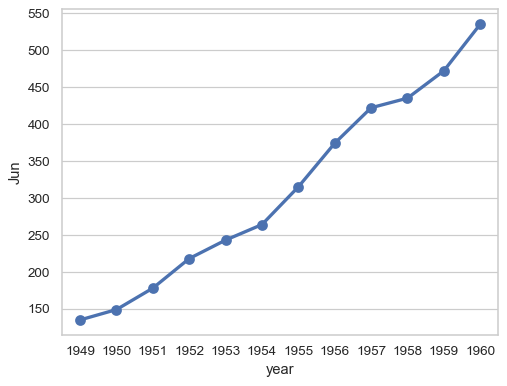Control the formatting of the categorical variable as it appears in the tick labels:

```sns.pointplot(flights_wide["Jun"], formatter=lambda x: f"'{x % 1900}")
```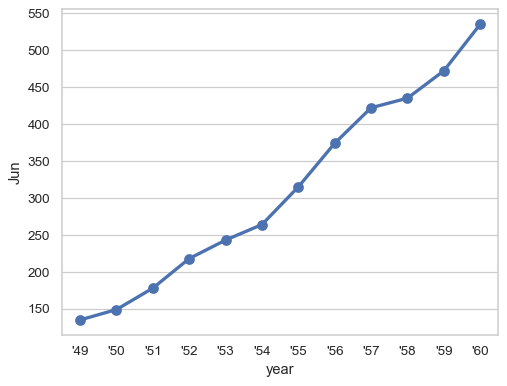Or preserve the native scale of the grouping variable:

```ax = sns.pointplot(flights_wide["Jun"], native_scale=True)
ax.plot(1955, 335, marker="*", color="r", markersize=10)
```